# Coherent sheaf

(diff) ← Older revision | Latest revision (diff) | Newer revision → (diff)

on a ringed space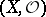A sheaf of modules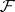over a sheaf of ringswith the following properties: 1)is a sheaf of finite type, that is, it is locally generated over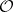by a finite number of sections; and 2) the kernel of any homomorphism of sheaves of modules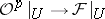over an open set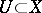is a sheaf of finite type. If in an exact sequenceof sheaves of-modules two of the three sheaves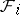are coherent, then the third is coherent as well. If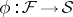is a homomorphism of coherent sheaves of-modules, then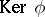,,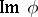are also coherent sheaves. Ifand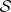are coherent, then so are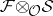and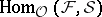.

A structure sheafis called a coherent sheaf of rings ifis coherent as a sheaf of modules over itself, which reduces to condition 2). Ifis a coherent sheaf of rings, then a sheaf of-modulesis coherent if and only if every point of the spacehas a neighbourhoodover which there is an exact sequence of sheaves of-modules: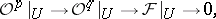. Furthermore, under this condition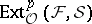is coherent for any coherent sheaves,and for all(see ).

The fundamental classes of ringed spaces with a coherent structure sheafare: analytic spaces over algebraically closed fields , Noetherian schemes and, in particular, algebraic varieties . A classical special case is the sheafof germs of holomorphic functions in a domain of; the statement that it is coherent is known as the Oka coherence theorem , . The structure sheaf of a real-analytic space is not coherent, in general.

How to Cite This Entry:
Coherent sheaf. Encyclopedia of Mathematics. URL: http://encyclopediaofmath.org/index.php?title=Coherent_sheaf&oldid=16458
This article was adapted from an original article by A.L. Onishchik (originator), which appeared in Encyclopedia of Mathematics - ISBN 1402006098. See original article